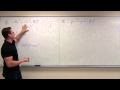Course

Intermediate Algebra by Leonard

5

(19)

Certificate

This course is free today and always will be, from start to finish you will pay nothing.Teacher

Leonard

This Channel is dedicated to quality mathematics education. It is absolutely FREE so Enjoy! Videos are organized in playlists and are course specific.

Course content preview

2h17m

0h54m

0h53m

1h39m

1h23m

1h05m

2h28m

0h38m

0h39m

1h42m

1h08m

1h10m

1h16m

0h57m

0h49m

0h40m

1h41m

0h46m

1h21m

Intermediate Algebra Lecture 8.4: An Introduction to Direct and Inverse Variation

0h46mWatch the 1st video of the course

Do not worry, it is free!

Course content2h17m

Intermediate Algebra Lecture 6.1: Factoring the Greatest Common Factor (GCF)

Intermediate Algebra Lecture 6.1: Factoring the Greatest Common Factor (GCF)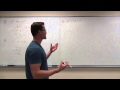0h54m

Intermediate Algebra Lecture 6.2: Factoring Polynomials (Trinomials) With a = 1

Intermediate Algebra Lecture 6.2: Factoring Polynomials (Trinomials) With a = 1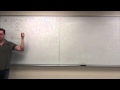0h53m

Intermediate Algebra Lecture 6.4: Factoring Polynomials (Trinomials) in General

Intermediate Algebra Lecture 6.4: Factoring Polynomials (Trinomials) in General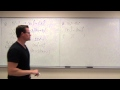1h39m

Intermediate Algebra Lecture 6.5: Factoring Binomials (Difference of Squares/Cubes)

Intermediate Algebra Lecture 6.5: Factoring Binomials (Difference of Squares/Cubes and Sum of Cubes)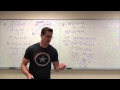1h23m

Intermediate Algebra Lecture 6.6: Solving Equations by Factoring

Intermediate Algebra Lecture 6.6: Solving Equations by Factoring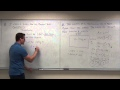1h05m

Intermediate Algebra Lecture 6.7: Using Factoring to Solve Word/Application Problems

Intermediate Algebra Lecture 6.7: Using Factoring to Solve Word/Application Problems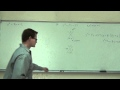2h28m

Intermediate Algebra Lecture C.1: A BRIEF Review of Solving Equations and Factoring

Intermediate Algebra Lecture C.1: A BRIEF Review of Solving Equations and Factoring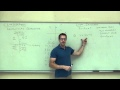0h38m

Intermediate Algebra Lecture C.3: A BRIEF Review of Graphing

Intermediate Algebra Lecture C.3: A BRIEF Review of Graphing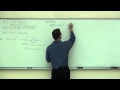0h39m

Intermediate Algebra Lecture C.4: A BRIEF Review of Polynomial Mathematics

Intermediate Algebra Lecture C.4: A BRIEF Review of Addition, Subtraction, Multiplication, Division of Polynomials1h42m

Intermediate Algebra Lecture 7.1: Defining and Simplifying Rational Expressions

Intermediate Algebra Lecture 7.1: Defining and Simplifying Rational Expressions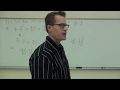1h08m

Intermediate Algebra Lecture 7.2: How to Multiply and Divide Rational Expressions

Intermediate Algebra Lecture 7.2: How to Multiply and Divide Rational Expressions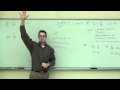1h10m

Intermediate Algebra Lecture 7.3: Finding LCD and Equivalent Rational Expressions

Intermediate Algebra Lecture 7.3: Finding Lowest Common Denominator and Equivalent Rational Expressions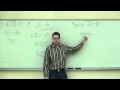1h16m

Intermediate Algebra Lecture 7.4: Adding and Subtracting Rational Expressions

Intermediate Algebra Lecture 7.4: Adding and Subtracting Rational Expressions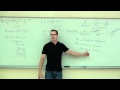0h57m

Intermediate Algebra Lecture 7.5: Solving Rational Equations (Equations with Rational Expressions)

Intermediate Algebra Lecture 7.5: Solving Rational Equations (Equations with Rational Expressions)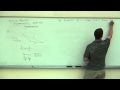0h49m

Intermediate Algebra Lecture 7.6: Using Proportions and Rational Methods in Problem Solving

Intermediate Algebra Lecture 7.6: Using Proportions and Rational Methods in Problem Solving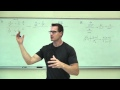0h40m

Intermediate Algebra Lecture 7.7: Introducing and Simplifying Complex Fractions

Intermediate Algebra Lecture 7.7: Introducing and Simplifying Complex Fractions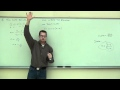1h41m

Intermediate Algebra Lecture 8.1: Graphing Lines. Equations of Parallel and Perpendicular Lines

Intermediate Algebra Lecture 8.1: Graphing Linear Functions. Equations of Parallel and Perpendicular Lines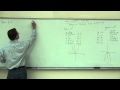0h46m

Intermediate Algebra Lecture 8.2: An Introduction to Non-Linear Functions

Intermediate Algebra Lecture 8.2: An Introduction to Non-Linear Functions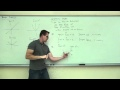1h21m

Intermediate Algebra Lecture 8.3: Study of Piecewise Functions and Basic Translations of Graphs

Intermediate Algebra Lecture 8.3: Study of Piecewise Functions and Basic Translations of Graphs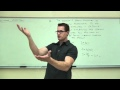0h46m

Intermediate Algebra Lecture 8.4: An Introduction to Direct and Inverse Variation

Intermediate Algebra Lecture 8.4: An Introduction to Direct and Inverse Variation